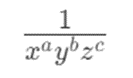# How to express the convex formula of 1/x^a*y^b*z^c in cvx?

I want to express, where x y and z are optimization variables, and a b c are constants.

Assuming a,b,\ldots x, y, \ldots are all positive then t\geq \frac{1}{x^ay^b\cdots} is equivalent with \log(t)+a\log(x)+b\log(y)+\cdots\geq 0.

If a,b,\ldots are natural numbers then you could also use geo_mean or prod_inv.

1 Like